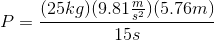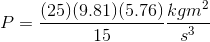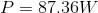# AP Physics C: Mechanics : Power

## Example Questions

### Example Question #5 : Work, Energy, And Power

When riding your bicycle, you have a maximum power output of 500W. As you approach a hill, you shift into first gear, applying a torque of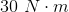to the gears. Assuming you produce 50% of your maximum power output, what is the angular velocity of the gears, in radians per second?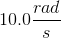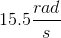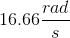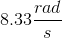Explanation:

Power is determined by calculating the work output per unit time. In this case, power will be the product of torque and angular velocity: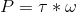We are given values for our torque and our power output, allowing us to solve for the angualr velocity. Keep in mind that the power output is going to be 50% of the maximum.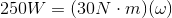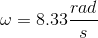### Example Question #6 : Work, Energy, And Power

A crane lifts a crate with a mass of 50kg. The crate is raised at a constant velocity for ten seconds and and moves a vertical distance upwards of 20m. What power is being supplied to the crane during this time?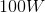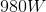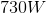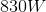Explanation:

For this problem we can calculate the power as the product of force and velocity: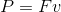First, we need to find the velocity: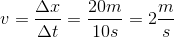Our force will be equal to the weight of the crate: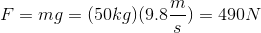Now, we can solve for power: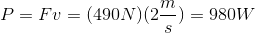### Example Question #7 : Work, Energy, And Power

A person is moving boxes up the stairs in their new home. They have two identical boxes, with same the size and mass. The first box is easy to carry up the stairs. When moving the second box, the person is more tired and moves more slowly. Which statement accurately describes the work and power between the two trials?

Work increases; power decreases

Work decreases; power stay the same

Work increases; power increases

Work remains the same; power decreases

Work remains the same; power increases

Work remains the same; power decreases

Explanation:

Because the boxes are the same mass and are moving the same distance, the work done will remain the same between the two instances. Work does not depend on time: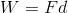However, when carrying the second box, the person moves more slowly. The overall time increases, which leads to a decrease in power.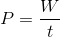### Example Question #8 : Work, Energy, And Power

What power is required to lift a 25kg box 5.76m off the ground in fifteen seconds?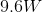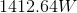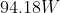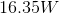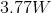Explanation:

The definition of power is:The work done on the box to lift it is required in order to overcome the force of gravity. If we can find the force of gravity on the object, we can calculate the net force. The force of gravity on any object near the Earth's surface is: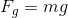The definition of work is: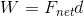We can substitute the force of gravity for the net force, resulting in the equation: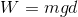Substituting this into our power equation, we find: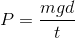Plugging in our given values and constants, we find: